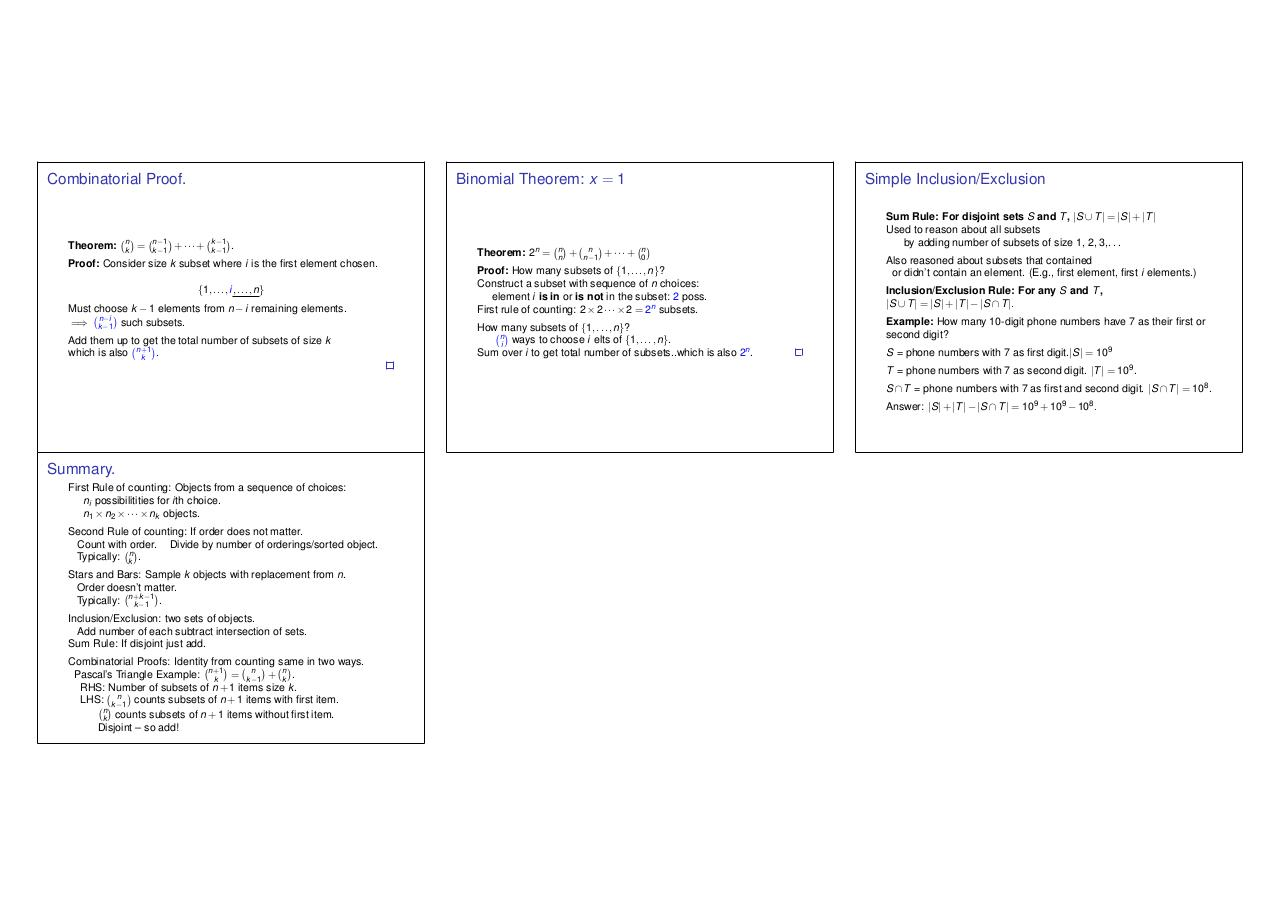# lec 15.6up.pdfPage 1 2 3 4 5 6 7

#### Text preview

Combinatorial Proof.

Theorem:

n
k

=

n 1
k 1

Binomial Theorem: x = 1

+···+

k 1
k 1

.

Proof: Consider size k subset where i is the first element chosen.
{1, . . . , i, . . . , n}

Must choose k 1 elements from n
=) kn 1i such subsets.

i remaining elements.

Add them up to get the total number of subsets of size k
1
which is also n+
k .

Theorem: 2n =

n
n

+

n
n 1

+···+

Simple Inclusion/Exclusion

n
0

Proof: How many subsets of {1, . . . , n}?
Construct a subset with sequence of n choices:
element i is in or is not in the subset: 2 poss.
First rule of counting: 2 ⇥ 2 · · · ⇥ 2 = 2n subsets.

How many subsets of {1, . . . , n}?
n
i ways to choose i elts of {1, . . . , n}.
Sum over i to get total number of subsets..which is also 2n .

Sum Rule: For disjoint sets S and T , |S [ T | = |S| + |T |
Used to reason about all subsets
by adding number of subsets of size 1, 2, 3,. . .
Also reasoned about subsets that contained
or didn’t contain an element. (E.g., first element, first i elements.)
Inclusion/Exclusion Rule: For any S and T ,
|S [ T | = |S| + |T | |S \ T |.

Example: How many 10-digit phone numbers have 7 as their first or
second digit?
S = phone numbers with 7 as first digit.|S| = 109
T = phone numbers with 7 as second digit. |T | = 109 .

S \ T = phone numbers with 7 as first and second digit. |S \ T | = 108 .

Summary.
First Rule of counting: Objects from a sequence of choices:
ni possibilitities for ith choice.
n1 ⇥ n2 ⇥ · · · ⇥ nk objects.

Second Rule of counting: If order does not matter.
Count with order. Divide by number of orderings/sorted object.
Typically: kn .
Stars and Bars: Sample k objects with replacement from n.
Order doesn’t matter.
k 1
Typically: n+
k 1 .
Inclusion/Exclusion: two sets of objects.
Add number of each subtract intersection of sets.
Sum Rule: If disjoint just add.
Combinatorial Proofs: Identity from counting same in two ways.
1
Pascal’s Triangle Example: n+
= k n 1 + kn .
k
RHS: Number of subsets of n + 1 items size k .
LHS: k n 1 counts subsets of n + 1 items with first item.
n
k counts subsets of n + 1 items without first item.